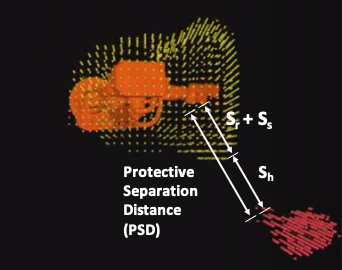# How Collaborative is your Robot? A Practical Approach — Veo Roboticswhere:

S is the minimum distance, in mm;

K is a parameter, in mm/sec, derived from data on approach speeds of the body or parts of the body;

T is the overall system stopping performance in seconds;

C is the intrusion distance, in mm.

Let’s call this distance S the Minimum Distance to Hazard, or MDH. If we generalize the above equation to make the robot the “hazardous machinery”, we see a correspondence between the MDH and PSD equations. The MDH calculation consists of two terms, which correspond to terms in the equation for the PSD calculation when safeguarding with SSM.

First the K x T term in the MDH calculation corresponds directly to S
h
, the contribution attributable to the human’s change in location, and the intrusion distance C corresponds identically to its counterpart in the PSD equation. The S
s
and S
r
terms are effectively zero in the MDH calculation because it assumes that the hazard itself does not move. The error terms Z
d
and Z
r
from the PSD calculation are not called out explicitly in the MDH calculation, but since they are constants, we can consider them subsumed as part of the intrusion distance C.

But the robot is moving, so how do we “map” it to the stationary machinery of ISO 13855? The way to do this is to introduce a Robot Future Cloud (RFC), which is all the space the robot could occupy by the time we have detected a PSD violation. We calculate the RFC to include a window of time before a PSD violation where the robot will continue to move through its planned trajectory, which may involve acceleration or deceleration.

The range of motion attributable to this pre-stop time window is calculated using the robot’s pose, the robot’s current load, the robot’s current velocity, a description of the robot dynamics and kinematics, any controller-enforced limits on robot joint velocity, and a bound on the time it takes for the sensing system (e.g. Veo FreeMove®) to issue a PSD violation signal and the time it takes the robot controller to acknowledge such a signal. The result of all this is a range of robot joint values that are attainable before the robot comes to a stop.

The range of joint values needs to be converted to a 3D space. This is done by comprehensively sampling the space of possible joint values and marking the space occupied by the robot’s links at these joint value samples. The result is an RFC representing all the 3D physical space the robot could possibly occupy before the sensing system assumes it could stop it. In terms of the PSD equation, the RFC is capturing the two terms missing in the MDH equation, S
s
and S
r
, and “incorporates” into the MDH calculation the fact that the robot is, in fact, moving.